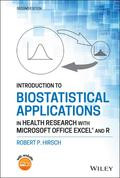John Wiley & Sons Introduction to Biostatistical Applications in Health Research with Microsoft Office Excel and RThe second edition of Introduction to Biostatistical Applications in Health Research delivers a thor.. Product #: 978-1-119-72259-5 Regular price: \$114.02 \$114.02 Auf Lager

# Introduction to Biostatistical Applications in Health Research with Microsoft Office Excel and R2. Auflage April 2021
640 Seiten, Hardcover
Wiley & Sons Ltd

ISBN: 978-1-119-72259-5
John Wiley & Sons

Jetzt kaufen

### Preis: 122,00 €

Preis inkl. MwSt, zzgl. Versand

The second edition of Introduction to Biostatistical Applications in Health Research delivers a thorough examination of the basic techniques and most commonly used statistical methods in health research. Retaining much of what was popular with the well-received first edition, the thoroughly revised second edition includes a new chapter on testing assumptions and how to evaluate whether those assumptions are satisfied and what to do if they are not.

The newest edition contains brand-new code examples for using the popular computer language R to perform the statistical analyses described in the chapters within. You'll learn how to use Excel to generate datasets for R, which can then be used to conduct statistical calculations on your data.

The book also includes a companion website with a new version of BAHR add-in programs for Excel. This new version contains new programs for nonparametric analyses, Student-Newman-Keuls tests, and stratified analyses. Readers will also benefit from coverage of topics like:
* Extensive discussions of basic and foundational concepts in statistical methods, including Bayes' Theorem, populations, and samples
* A treatment of univariable analysis, covering topics like continuous dependent variables and ordinal dependent variables
* An examination of bivariable analysis, including regression analysis and correlation analysis
* An analysis of multivariate calculations in statistics and how testing assumptions, like assuming Gaussian distributions or equal variances, affect statistical outcomes

Perfect for health researchers of all kinds, Introduction to Biostatistical Applications in Health Research also belongs on the bookshelves of anyone who wishes to better understand health research literature. Even those without a great deal of mathematical background will benefit greatly from this text.

Preface

PART ONE: Basic Concepts

1.1.Properties of Chance

1.2.Combinations of events

1.2.1 Intersections

1.2.2 Unions

1.3.Bayes' theorem

2.Describing Populations

2.1.Types of data

2.2.Describing distributions graphically

2.2.1. Graphing discrete data

2.2.2. Graphing continuous data

2.2.3. Frequency polygon

2.3.Describing distributions mathematically

2.3.1. Parameter of location

2.3.2. Parameter of dispersion

2.4 Taking chance into account

2.4.1 Standard normal distribution

3.Examining Samples

3.1.Nature of samples

3.2.Estimation

3.2.1 Point estimates

3.2.2 The sampling distribution

3.2.3 Interval estimates

3.3.Hypothesis testing

PART TWO: Univariable Analysis

4.Univariable Analysis of a Continuous Dependent Variable

4.1.Student's t distribution

4.2.Interval estimation

4.3.Hypothesis testing

5.Univariable Analysis of an Ordinal Dependent Variable

5.1 Nonparametric methods

5.2 Estimation

5.3 Wilcoxon signed-rank test

5.4 Statistical power of nonparametric tests

6.Univariable Analysis of a Nominal Dependent Variable

6.1.Distributions of nominal data

6.2.Point estimates

6.2.1 Proportions

6.2.2 Rates

6.3.Sampling distributions

6.3.1 Binomial distribution

6.3.2 Poisson distribution

6.4.Interval estimation

6.5.Hypothesis testing

PART THREE: Bivariable Analysis

7.Bivariable Analysis of a Continuous Dependent Variable

7.1.Continuous independent variable

7.1.1 Regression analysis

7.1.2 Correlation analysis

7.2.Ordinal independent variable

7.3.Nominal independent variable

7.3.1 Estimating the difference between groups

7.3.2 Taking chance into account

8.Bivariable Analysis of an Ordinal Dependent Variable

8.1.Ordinal independent variable

8.2.Nominal independent variable

9.Bivariable Analysis of a Nominal Dependent Variable

9.1.Continuous independent variable

9.1.1 Estimation

9.1.2 Hypothesis testing

9.2.Nominal independent variable

9.2.1 Dependent variable not affected by time: Unpaired design

9.2.2 Dependent variable not affected by time: Paired design

9.2.3 Dependent variable affected by time

PART FOUR: Multivariable Analysis

10.Multivariable Analysis of a Continuous Dependent Variable

10.1.Continuous independent variables

10.1.1 Multiple regression analysis

10.1.2 Multiple correlation analysis

10.2.Nominal independent variables

10.2.1 Analysis of variance

10.2.2 Posterior tests

10.3.Continuous and nominal independent variables

10.3.1 Indicator ("dummy") variables

10.3.2 Interaction variables

10.3.3 General linear model

11.Multivariable Analysis of an Ordinal Dependent Variable

11.1.Nonparametric ANOVA

11.2.Posterior testing

12.Multivariable Analysis of a Nominal Dependent Variable

12.1.Continuous and/or nominal independent variables

12.1.1 Maximum likelihood estimation

12.1.2 Logistic regression analysis

12.1.3 Cox regression analysis

12.2.Nominal independent variables

12.2.1 Stratified analysis

12.2.2 Life table analysis

13. Testing Assumptions

13.1Continuous dependent variables

13.1.1 Assuming a Gaussian distribution

13.1.2 Transforming dependent variables

13.1.3 Assuming equal variances